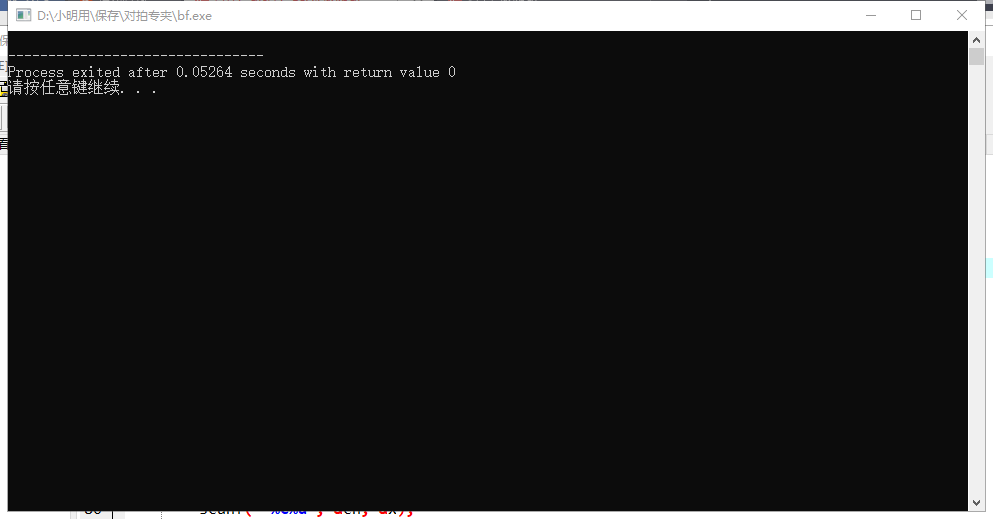# 对拍过程讲解

2019/08/23 11:31

$1.$ 当你在打比赛（或考试）时，想出了一个高分代码，但是不保证正确性。

$2.$ 当你在某$\text{OJ}$上做某题$\text{WA}$了，但是你死活调试不出来，而你又没有数据或者可以下载数据但是数据过大无法找出原因

提前声明一下：下面的几个程序最好不要用快读。因为用了$\text{freopen}$，不用快读时编译程序程序会自动结束，但是用了快读程序就不会自动结束。这样导致对拍也无法进行。（快写还是可以用的）$\text{Part 1}$对拍具体过程

$\text{P.S: }这一部分只对具体过程做一个讲解，代码见\text{Part 2}$

$1.$对你的程序进行操作。 你已经写好了你的程序了，那么现在你要在你的主函数开头加这些话：

freopen("data.in", "r", stdin);
freopen("data.ans", "w", stdout);

$2.$对标程进行操作。

freopen("data.in", "r", stdin);
freopen("data.out", "w", stdout);

$3.$制造数据。

freopen("data.in", "w", stdout);

$4.$写对拍程序。

$\text{sol.exe}$

$\text{bf.exe}$

$\text{random.exe}$

$\text{Part 2}$ 对拍过程程序模板$接下来提供一些模板~$1. $对拍程序模板 ①$\text{Windows}$对拍模板 #include <cstdio> #include <ctime> #include <cstdlib> using namespace std; int main() { for (int T = 1; T <= 100; T++) { system("random.exe"); double st = clock(); system("sol.exe"); double ed = clock(); system("bf.exe"); if (system("fc data.out data.ans")) { printf("Wrong Answer!\n"); return 0; } printf("Accepted 测试点#%d 耗时%.0lf\n", T, ed - st); } return 0; } 注意，两个$\text{clock}$函数之间必须是$\text{system("sol.exe")}$。这里就跟前缀和一样，第一个$\text{clock()}$返回从运行$\text{sol.exe}$到开始运行程序的时间，第二个$\text{clock()}$返回从运行完毕$\text{sol.exe}$到开始运行程序的时间。两者相减即是$\text{sol.exe}$的运行时间。所以对于这三个$\text{system}$的顺序也不用死记硬背，先造数据嘛$\text{(random.exe)}$，然后再来计时$\text{(sol.exe)}$，最后运行标程$\text{(bf.exe)}$。先运行标程再来计时也成。 ②$\text{Linux}$对拍模板 其实和$\text{Windows}$对拍模板总体上没有什么区别...只不过要把$\text{fc}$命令改成$\text{diff}$即可。$2. \text{random}$程序模板 首先提供一个基础模板： #include<cstdlib> #include<ctime> using namespace std; inline int random (int n) { return (long long)rand() * rand() % n; } int main() { srand((unsigned)time(0)); //...具体内容... return 0; } 对于任何的$\text{random}$程序，这些语句是必加的。 关于这个程序的解释，我们来看看蓝书的句子：$\text{rand}$函数会返回一个$0$到$\text{RAND}$-$\text{MAX}$的值。$\text{srand(seed)}$函数接受$\text{unsigned int}$类型的参数$\text{seed}$，以$\text{seed}$为“随机种子”，$\text{rand}rand$函数基于线性同余递推式生成随机数，“随机种子”相当于计算线性同余时的一个初始参数。 如果不执行$\text{srand}$函数，则种子默认为$1$。 当种子确定后，接下来产生的随机数列就是固定的，所以这种随机方法也被称为“伪随机”。因此，一般在罪及数据生成程序$\text{main}$函数的开头，用当前系统时间作为随机种子。 头文件$\text{ctime(time.h)}$包含$\text{time}$函数，调用$\text{time(0)}$可以返回从$1970$年$1$月$1$日$0$时$0$分$0$秒（$\text{Unix}$纪元）到现在的秒数。执行$\text{srand((unsigned)time(0))}$即可初始化随机种子。 一般来说用$\text{rand()}$函数就可以随机生成一个数了。 至于为什么蓝书单独写一个$\text{random}$函数...据说是“综合考虑了操作系统和编译器环境的差异，对$\text{int}$范围内的$\text{n}n$均能正常工作”。 引用$\text{random(x)}$会返回$0$~$\text{x} - 1$任意一个数字。但是大多数的题中数的范围在$1$~$\text{x}$，所以还要再加个$1$所以要生成一个$1$~$\text{x}$的数通常写： int y = random(x) + 1 基础模板就讲到这里，接下来免费再提供$6$个模板哦~(*^▽^*)$1. $随机生成整数序列。 #include<cstdlib> #include<cstdio> #include<ctime> using namespace std; const int N = 100010; int a[N]; inline int random (int n) { return (long long)rand() * rand() % n; } int main() { srand((unsigned)time(0)); int n = random(100000) + 1; int m = 100000000; for (int i = 1; i <= n; i++) { a[i] = random(2 * m + 1) - m; } for (int i = 1; i <= n; i++) printf("%d ", a[i]); return 0; }/* 此模版会生成n<=10^5个绝对值在10^9之内的整数*/ 以上面这个模板为例，简单讲解一下随机数生成程序的具体意思 首先加上$\text{srand(unsigned)time(0)}$这一句（必加！！必加！！必加！！） 由于要生成$\text{n}$个数，所以首先应该生成一个整数$\text{n}$。由于要求生成不超过$10^5$个数，所以$\text{n = random(100000) + 1}$。（之所以加$1$是因为$\text{random}$返回的值在$0$~$99999$之间，所以应该加一个$1$）。 接下来就开始生成这$\text{n}$个数了。由于绝对值在$10^9$之内，而$\text{random}$又不能返回负数，所以可以考虑把绝对值的范围“向后移动$10^9$个单位”，范围变成了$0$~$2\times 10^9$。再把生成的数减去$10^9$就可以保证数据范围在$-10^9$~$10^9$之间了。 如果想要生成小数，可以先随机生成一个整数，然后除以$10$的次幂即可。 下面的模板同理，就不做讲解啦~（但是那个随机生成图蒟蒻没有搞懂...）$2.$随机生成区间列。 #include<cstdlib> #include<ctime> #include<iostream> #include<cstdio> using namespace std; int m,n; inline int random (int n) { return (long long)rand() * rand() % n; } int main() { srand((unsigned)time(0)); scanf("%d%d", &m, &n); for (int i = 1; i <= m; i++) { int l = random(n) + 1; int r = random(n) + 1; if (l > r) swap(l, r); printf("%d %d\n", l, r); } return 0; }/* 此模版会生成m个[1,n]的子区间，这些区间可作为数据结构题目的操作序列*/$3. $随机生成树。 #include<cstdlib> #include<ctime> #include<cstdio> using namespace std; int n; inline int random (int n) { return (long long)rand() * rand() % n; } int main() { srand((unsigned)time(0)); scanf("%d", &n); printf("%d\n", n); for (int i = 2; i <= n; i++) { //从2~n之间的每个点i向1~i-1之间的点随机连一条边 int fa = random(i - 1) + 1; int val = random(1000000000) + 1; printf("%d %d %d\n", fa, i, val); } return 0; }/* 此模版会随机生成一棵n个点的树，用n个点n-1条边的无向图的形式输出，每条边附带一个10^9以内的正整数权值。*/$4.$随机生成图。 #include<bits/stdc++.h> using namespace std; pair<int, int> e;//保存数据 map<pair<int, int>, bool> h;//防止重边 inline int random (int n) { return (long long)rand() * rand() % n; } int main() { srand((unsigned)time(0)); //先生成一棵树，保证连通 int n = random(100000) + 1; int m = random(100000) + 1; printf("%d %d\n", n, m); for (int i = 1; i < n; i++) { int fa = random(i) + 1; e[i] = make_pair(fa, i + 1); h[e[i]] = h[make_pair(i + 1, fa)] = 1; } //再生成剩余的m - n + 1条边 for (int i = n; i <= m; i++) { int x, y; do { x = random(n) + 1, y = random(n) + 1; } while(x == y || h[make_pair(x, y)]); e[i] = make_pair(x, y); h[e[i]] = h[make_pair(y, x)] = 1; } //随机打乱，输出 random_shuffle(e + 1, e + m + 1); for (int i = 1; i <= m; i++) printf("%d %d\n", e[i].first, e[i].second); return 0; }$5.$随机生成链 #include <cstdio> #include <ctime> #include <cstdlib> #include <algorithm> using namespace std; #define ll long long const int N = 1e5 + 5; pair <int, int> e[N]; int a[N]; bool p[N]; inline int random (int x) { return (ll)rand() * rand() % x; } int main () { srand((unsigned)time(0)); int n = random(100000) + 1; printf("%d\n", n); for (int i = 1; i <= n; i++) { int x = random(n) + 1; while (p[x]) { x = random(n) + 1; } p[x] = 1; a[i] = x; } for (int i = 1; i < n; i++) e[i] = make_pair(a[i], a[i + 1]); random_shuffle(e + 1, e + n);//随机打乱 for (int i = 1; i < n; i++) { int z = random(1000000) + 1; printf("%d %d %d\n", e[i].first, e[i].second, z); } return 0; }$6.$随机生成菊花图 #include <cstdio> #include <ctime> #include <cstdlib> #include <algorithm> using namespace std; #define ll long long const int N = 1e5 + 5; pair <int, int> e[N]; bool p[N]; inline int random (int x) { return (ll)rand() * rand() % x; } int main () { srand((unsigned)time(0)); int n = random(100000) + 1; printf("%d\n", n); int root = random(n) + 1; p[root] = 1; for (int i = 1; i < n; i++) { int x = random(n) + 1, y = random(2); //y用来表示是否交换这条边的两个顶点输入顺序 while (p[x]) x = random(n) + 1; p[x] = 1; if (y) e[i] = make_pair(root, x); else e[i] = make_pair(x, root); } random_shuffle(e + 1, e + n); for (int i = 1; i < n; i++) { int z = random(1000000) + 1; printf("%d %d %d\n", e[i].first, e[i].second, z); } return 0; } 注意，你的程序有可能是无法$\text{AC}$的，但是有可能对拍无法将你的程序$\text{hack}$掉。 因为数据是随机生成的。而题目往往可能会想某些你没有注意的方向出数据。所以你需要自己单独制造更特殊的数据。 有三种数据可以对树、图进行极端情况下的测试：$1.$链形数据--有很长的直径。$2.$菊花形数据--有度数很大的节点。$3.$蒲公英形数据。树的一部分是链，另一部分是菊花。$\text{Part 3}$对拍中的特殊情况处理 假设你在做$\text{UVA}$上的一道题，这是你发现你死活卡不过$\text{UVA}$上的毒瘤数据，于是你决定对拍来找数据。 这本来是个很简单的过程，但是你发现这道题是有多组输出的！ 于是你愣在原地，不知所措 我们平常的对拍用的是$\text{fc}$命令，$\text{fc}$通过比较两个文件的输出是否相同来判断你的答案是否正确。但是这道题已经有多组解了，那么直接对比答案肯定是不行的，那么该怎么办呢？ 一种方法是写$\text{SPJ}$，不过要下载什么东西来着，感觉很麻烦。 还有一种方法就是在$\text{C++}$内部进行$\text{SPJ}$，那么应该怎么做呢？给$10$秒钟的时间思考一下... 既然$fc$是通过对比文件输出是否相同来判断你的答案是否正确的，那么我们就可以尝试写一个程序，通过判断$\text{data.out}$与$\text{data.ans}$的内容是否满足题目中条件，如果满足条件就把一个特定的值输出到第三个输出文件中（比如说$1$），最后让第三个文件与一个内容为$1$的文件对比，不相同则输出$\text{"Wrong Answer!"}$。 例子：UVA529 我写的$\text{SPJ}$程序: #include <bits/stdc++.h> using namespace std; int x, tot1, tot2; int main() { freopen("data.out", "r", stdin); while (~scanf("%d", &x)) tot1++; freopen("data.ans", "r", stdin); while (~scanf("%d", &x)) tot2++; freopen("data.txt", "w", stdout); printf("%d\n", tot1 == tot2 ? 1 : 0); return 0; } 这种方法不但方便，在$\text{CSP}$考场上也是可以用滴！（毕竟在$\text{CSP}$考场上泥不可能下载一个软件来写$\text{SPJ}$的） 关于对拍的讲解就到这里啦~有疑问可以在下方评论区提出哦~(*^▽^*)。 ---------------------------------------------------------------------------------------------------------------------------------------------- 感谢@$WYXkk$对本文内容进行修正。（$update:2019.9.8$） 补充了一种在对拍中遇到的特殊情况，感谢$@ThinkofBlank$对本蒟蒻的指点qwq。（$update:2019.11.11$） 补充了随机生成链和菊花图的模板。（$update:2019.11.19\$）### 作者的其它热门文章

0
0 收藏

0 评论
0 收藏
0